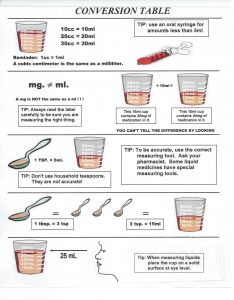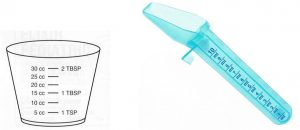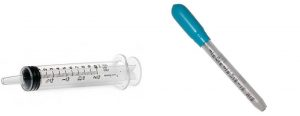### 3.4. Metric system

Metric system – a decimal system of standard weights and measures using the:

• milligram (mg);
• gram (g or gm);
• kilogram (kg);
• milliliter (ml);
• liter (l)… among others.

Note: a cubic centimeter (cc) is the same amount as a milliliter (ml) –or– 1 cc = 1 ml

Household system – a system based on common, though not standard, measuring devices:

• teaspoon and tablespoon can be different sizes.

Equivalents that must be learned:

•     1 tsp. = 5 cc = 5 ML
•     3 tsp. = 1 Tbsp = 15 cc = 15 ML = 1/2 OUNCE
•     2 Tbsp = 30 cc = 30 ML = 1 oz##### Measuring devices.Medication cap.                                                    SpoonOral syringe                                                  Oral dropper.